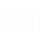Select Page

Rating analysis methods are based on a weighted scoring.

Weighted scoring is a method for ranking and evaluating objects of analysis based on several weighted (balancing) criteria. The evaluation criterion is an indicator, a measure, a measured characteristic.

The following methods of analysis are implemented:

• Weighted scoring - based on the values of all evaluation criteria
• Hamburg score - based on the estimated ratings of all evaluation criteria

For the evaluation criterion (further Indicator) the user defines:

 Property Description Normative The normative value of the indicator Weight Indicator weight Direction of improvement Direction of indicator improvement: better more (more) or better less (less)

## Weighted scoring

Self-weighted scoring based on the values of all evaluation criteria according to the methodology described below.

## Analysis Method

The user defines:

• Object of analysis
• Criteria for evaluation
• Criteria weights. The evaluation criterion that best fits your goals is given the highest weight, while less important ones are given a lighter weight. In this way, weights are assigned to all other criteria and their importance rating is formed.

By default, all criteria are given the same weight, which corresponds to equal importance.

The program calculates:

• The total sum of all weights of the evaluation criteria.
• Weight coefficient for each of the evaluation criteria, as a share of its weight in the total weights.
• The value of the synthetic criterion for each object of analysis, as the sum of the values of all evaluation criteria multiplied by their weight coefficients.

The value of a synthetic criterion does not make economic sense, but it makes it possible to characterize each object of analysis with a single number.

You can dynamically change the criteria and their weights, getting a flexible evaluation model, shaping it in accordance with your goals, priorities, and tasks.

In this way, you create a scoring system for assessing the objects of analysis, effectively calculating the total value of each of them.

Rating features:

• Complexity (integrality) - the rating includes data characterizing all the main criteria for evaluating the object of analysis;
• Objectivity - the rating does not operate with expert opinions, it uses only indicators and their weights.

Based on the value of the synthetic criterion, it is possible to carry out an ABC classification according to the classical scheme.

## Hamburg score

Weighted score based on the score ratings of all evaluation criteria.

The Hamburg score is an evaluation of something without discounts and concessions with the utmost exactingness. Phraseological turn, concerning the true system of values, free from momentary circumstances and selfish interests.

The evaluation rating is formed according to the algorithm:

IF [Direction of improvement] = more

THEN ([Indicator value] - MIN()) / ( MAX()-MIN() )

ELSE ( MAX() - [Indicator value]) / ( MAX()-MIN() )

Where:

MIN() - the minimum value of the indicator in the set, including the normative

MAX() - the maximum value of the indicator in the set, including the normative

This is followed by the calculation of the Synthetic Criterion Value according to the Estimated Rating Values ​​according to the analysis methods described above.

## Examples of calculation

Criteria Properties

||A | b | c | D | e | ||---|---:|---:|---:|---:| ||Parameter\Indicator | Reseller Sales Amount | Reseller Order Quantity | Reseller Freight Cost | Reseller Tax Amount | |1|Normal | \$50,000,000.00 | 60,000 | \$600,000.00 | \$2,000,000.00 | |2|Weight | 70% | 20% | 4%| 6% | |3|Direction of improvement | more | more | less | less |

Criteria values

||A | b | c | D | e | ||---:|---:|---:|---:|---:| ||Object of evaluation\Indicator | Reseller Sales Amount | Reseller Order Quantity | Reseller Freight Cost | Reseller Tax Amount | |1|Bikes |\$66,302,381.56|75,015|\$1,657,560.05|\$5,304,190.40 | |2|Components |\$11,799,076.66|49,027|\$294,977.15 |\$943,926.12 | |3|Clothing |\$1,777,840.84 |64,497|\$44,446.19 |\$142,227.25 | |4|Accessories|\$571,297.93 |25,839|\$14,282.52 |\$45,703.83 |

Rating calculation

||A | b | c | D | e | F | G | h | I | J | |---|---|---:|---:|---:|---:|---:|---:|---:|---:|- --:|---:| ||Object of evaluation|Reseller Sales Amount | WRSA | Reseller Order Quantity | WROQ | Reseller Freight Cost | W RFC |Reseller Tax Amount | W RTA | Value

[B]*[C]+[D]*[E]+[F]*[G]+[H]*[I]

| |1|Bikes |\$66,302,381.56|0.7|75,015|0.2|\$1,657,560.05|0.04|\$5,304,190.40 |0.06|46,811,224| |2|Components |\$11,799,076.66|0.7|49,027|0.2|\$294,977.15 |0.04|\$943,926.12 |0.06|8,337,594 | |3|Clothing |\$1,777,840.84 |0.7|64,497|0.2|\$44,446.19 |0.04|\$142,227.25 |0.06|1,267,699 | |4|Accessories |\$571,297.93 |0.7|25,839|0.2|\$14,282.52 |0.04|\$45,703.83 |0.06|408,390 |

where W - Weight - Weight coefficient

## User Calculations

User calculations for calculating the value of the synthetic criterion

• ratings generated by the program.

- - -Weighted scoring- - -

- - - the sum of the values of all evaluation criteria multiplied by their weighting coefficients- - - Hamburg score- - -

- - - the sum of the evaluation ratings of all evaluation criteria multiplied by their weighting coefficients- - - evaluation rating for "better more" indicators- - -- - - evaluation rating for "better less" indicators- - -Write US